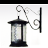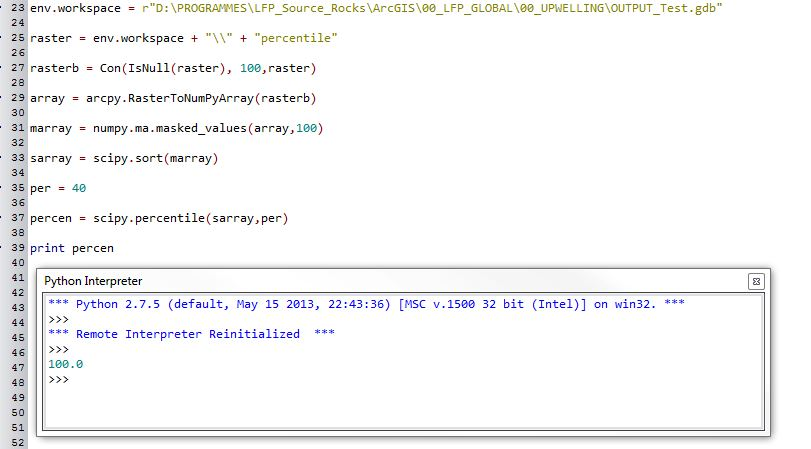# Excluding "NoData" in raster percentile calculation

3311
7
07-16-2015 07:31 AMOccasional Contributor II

Hi,

I'm trying to calculate percentiles out of a floating point raster.

It works BUT it includes the NoData in the calculation so I don't get what I need.

Is there a way to exclude the NoData from this calculation?

Many thanks

`env.workspace = r"D:\PROGRAMMES\LFP_Source_Rocks\ArcGIS\00_LFP_GLOBAL\00_UPWELLING\OUTPUT_Test.gdb"raster = env.workspace + "\\" + "percentile"array = arcpy.RasterToNumPyArray(raster)array = scipy.sort(array)per = 10a = scipy.percentile(array,per)print a`
Tags (5)
1 Solution

Accepted SolutionsOccasional Contributor II

Looks as if I finally managed to get it running. Code looks like this:

`env.workspace = r"D:\PROGRAMMES\LFP_Source_Rocks\ArcGIS\00_LFP_GLOBAL\00_UPWELLING\OUTPUT_Test.gdb"raster = env.workspace + "\\" + "percentile"array = arcpy.RasterToNumPyArray(raster,nodata_to_value=10000000)marray = numpy.ma.masked_values(array,10000000)sarray = scipy.sort(marray)per = 40percen = numpy.percentile(sarray.compressed(),(per))print percen`

7 RepliesOccasional Contributor II

Hi Toni,

The arcpy.RasterToNumPyArray tool has a parameter called 'nodata_to_value'.  This parameter allows you to set a value to assign to your input raster's nodata.  The default value is "None," which is probably what's messing up your calculations.

Tool help - ArcGIS Help (10.2, 10.2.1, and 10.2.2)

Cheers,

ToddOccasional Contributor II

Hi Todd,

I'm aware NoData can be assigned to a particular value. But, wouldn't that then "corrupt" the percentile calculation? as in this case there would be a lot of say value 1 (if for example I assign NoData to 1).

I'm working with global rasters with values present only 250km from the coast.

I think for the percentile calculation to be correct only the real list of values should be taken into account excluding the NoData values.

Or maybe I'm not quite right...

CheersbyMVP Esteemed ContributorOccasional Contributor II

Sorry Toni, you are correct in that you still have to deal with the noData in some way as to not taint your calculations - I thought you were just wondering if you could modify the noData.

You could probably use an array mask on your numpy array to exclude the values you don't want to include.

More info on how to do this here The numpy.ma module — NumPy v1.9 Manual

Cheers,

ToddOccasional Contributor II

Thanks Todd/Dan,

I'm trying to use numpy mask module. Not sure I'm getting the right syntax when calling the "NoData" (Null, NoData...) values.

I don't quite see how to do so in the documentation. This is how my code looks at the moment.

Any further help would be greatly appreciate it.

`env.workspace = r"D:\PROGRAMMES\LFP_Source_Rocks\ArcGIS\00_LFP_GLOBAL\00_UPWELLING\OUTPUT_Test.gdb"raster = env.workspace + "\\" + "percentile"array = arcpy.RasterToNumPyArray(raster)marray = numpy.ma.masked_values(array,NULL)sarray = scipy.sort(marray)per = 20a = scipy.percentile(sarray,per)print a`Occasional Contributor II

Hi again,

I've been trying also to set NoData to a value and then use "ma.masked_values" to exclude that value from the percentile calculation but it looks as if the value is not excluded.Occasional Contributor II

Looks as if I finally managed to get it running. Code looks like this:

`env.workspace = r"D:\PROGRAMMES\LFP_Source_Rocks\ArcGIS\00_LFP_GLOBAL\00_UPWELLING\OUTPUT_Test.gdb"raster = env.workspace + "\\" + "percentile"array = arcpy.RasterToNumPyArray(raster,nodata_to_value=10000000)marray = numpy.ma.masked_values(array,10000000)sarray = scipy.sort(marray)per = 40percen = numpy.percentile(sarray.compressed(),(per))print percen`